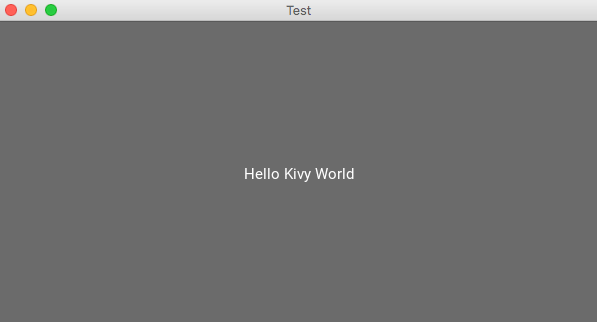# 七個Python必備的GUI庫

GUI(圖形用戶界面)，顧名思義就是用圖形的方式，來顯示計算機操作的界面，更加方便且直觀。

## 01. PyQt5

PyQt5由Riverbank Computing開發。基於Qt框架構建，是一個跨平臺框架，可以給各種平臺創建應用程序，包括：Unix、Windows、Mac OS。

PyQt將Qt和Python結合在一起。它不隻是一個GUI工具包。還包括瞭線程，Unicode，正則表達式，SQL數據庫，SVG，OpenGL，XML和功能完善的Web瀏覽器，以及許多豐富的GUI小部件集合。

```# 安裝PyQt5
pip install -i https://pypi.tuna.tsinghua.edu.cn/simple PyQt5```

```import sys
from PyQt5.QtWidgets import QApplication, QWidget, QLabel, QVBoxLayout

# 建立application對象
app = QApplication(sys.argv)
# 建立窗體對象
w = QWidget()
# 設置窗體大小
w.resize(500, 500)

# 設置樣式
w.layout = QVBoxLayout()
w.label = QLabel("Hello World!")
w.label.setStyleSheet("font-size:25px;margin-left:155px;")
w.setWindowTitle("PyQt5 窗口")
w.setLayout(w.layout)

# 顯示窗體
w.show()
# 運行程序
sys.exit(app.exec_())```https://riverbankcomputing.com/software/pyqt/intro

https://www.guru99.com/pyqt-tutorial.html

## 02. Tkinter

Tkinter是Python中最受歡迎的GUI庫之一。由於它簡單易學的語法，成為GUI開發初學者的首選之一。

Tkinter提供瞭各種小部件，例如標簽，按鈕，文本字段，復選框和滾動按鈕等。

```# 安裝tkinter
pip install -i https://pypi.tuna.tsinghua.edu.cn/simple tkinter```

```from tkinter import *
from tkinter import messagebox

def get_height():
# 獲取身高數據(cm)
height = float(ENTRY2.get())
return height

def get_weight():
# 獲取體重數據(kg)
weight = float(ENTRY1.get())
return weight

def calculate_bmi():
# 計算BMI系數
try:
height = get_height()
weight = get_weight()
height = height / 100.0
bmi = weight / (height ** 2)
except ZeroDivisionError:
messagebox.showinfo("提示", "請輸入有效的身高數據!!")
except ValueError:
messagebox.showinfo("提示", "請輸入有效的數據!")
else:
messagebox.showinfo("你的BMI系數是: ", bmi)

if __name__ == '__main__':
# 實例化object，建立窗口TOP
TOP = Tk()
TOP.bind("<Return>", calculate_bmi)
# 設定窗口的大小(長 * 寬)
TOP.geometry("400x400")
# 窗口背景顏色
TOP.configure(background="#8c52ff")
# 窗口標題
TOP.title("BMI 計算器")
TOP.resizable(width=False, height=False)
LABLE = Label(TOP, bg="#8c52ff", fg="#ffffff", text="歡迎使用 BMI 計算器", font=("Helvetica", 15, "bold"), pady=10)
LABLE.place(x=55, y=0)
LABLE1 = Label(TOP, bg="#ffffff", text="輸入體重(單位：kg):", bd=6,
LABLE1.place(x=55, y=60)
ENTRY1 = Entry(TOP, bd=8, width=10, font="Roboto 11")
ENTRY1.place(x=240, y=60)
LABLE2 = Label(TOP, bg="#ffffff", text="輸入身高(單位：cm):", bd=6,
LABLE2.place(x=55, y=121)
ENTRY2 = Entry(TOP, bd=8, width=10, font="Roboto 11")
ENTRY2.place(x=240, y=121)
font=("Helvetica", 20, "bold"))
BUTTON.grid(row=5, column=0, sticky=W)
BUTTON.place(x=115, y=250)
TOP.mainloop()```## 03. Kivy

Kivy是另一個開源的Python庫，最大的優點就是可以快速地編寫移動應用程序(手機)。

Kivy可以在不同的平臺上運行，包括Windows、Mac OS、Linux、Android、iOS和樹莓派。

```# 安裝kivy
pip install -i https://pypi.tuna.tsinghua.edu.cn/simple kivy```

```from kivy.app import App
from kivy.uix.button import Button

class TestApp(App):
def build(self):
return Button(text=" Hello Kivy World ")

TestApp().run()```## 04. wxPython

wxPython是一個跨平臺GUI的Python庫，可輕松創建功能強大穩定的GUI，畢竟是用C++編寫的～

```# 安裝wxPython
pip install -i https://pypi.tuna.tsinghua.edu.cn/simple wxPython```

```import wx

myapp = wx.App()
init_frame = wx.Frame(parent=None, title='WxPython 窗口')

init_frame.Show()
myapp.MainLoop()```## 05. PySimpleGUI

PySimpleGUI也是基於Python的GUI框架。可以輕松制作自定義的GUI。

Remi將應用程序的界面轉換為HTML，以便在Web瀏覽器中呈現。

```# 安裝PySimpleGUI
pip install -i https://pypi.tuna.tsinghua.edu.cn/simple PySimpleGUI```

```import PySimpleGUI as sg

layout = [[sg.Text("測試 PySimpleGUI")], [sg.Button("OK")]]
window = sg.Window("樣例", layout)
while True:
if event == "OK" or event == sg.WIN_CLOSED:
break
window.close()```## 06. PyGUI

PyGUI是一個以簡單API而聞名的GUI框架，減少Python應用與平臺底層GUI之間的代碼量。

Python2和Python3，都是可以支持的。

https://www.cosc.canterbury.ac.nz/greg.ewing/python_gui/

https://realpython.com/pysimplegui-python/

## 07. Pyforms

Pyforms是用於開發GUI應用程序的一個跨平臺框架。pyforms是一個Python2.7/3.x跨環境圖形應用開發框架，模塊化和代碼復用可以節省大量工作。

```# 安裝PyFroms
pip install -i https://pypi.tuna.tsinghua.edu.cn/simple PyFroms```